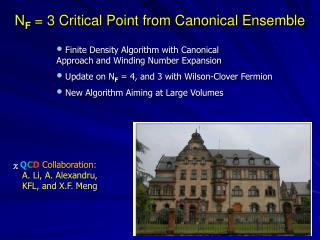DownloadDownload PresentationN F = 3 Critical Point from Canonical Ensemble

# N F = 3 Critical Point from Canonical Ensemble

Download Presentation## N F = 3 Critical Point from Canonical Ensemble

- - - - - - - - - - - - - - - - - - - - - - - - - - - E N D - - - - - - - - - - - - - - - - - - - - - - - - - - -
##### Presentation Transcript

1. NF = 3 Critical Point from Canonical Ensemble • Finite Density Algorithm with Canonical Approach and Winding Number Expansion • Update on NF = 4, and 3 with Wilson-Clover Fermion • New Algorithm Aiming at Large Volumes χQCDCollaboration: A. Li, A. Alexandru, KFL, and X.F. Meng

2. A Conjectured Phase Diagram

3. Canonical partition function

4. Canonical ensembles Fourier transform Canonical approach K. F. Liu, QCD and Numerical Analysis Vol. III (Springer,New York, 2005),p. 101. Andrei Alexandru, Manfried Faber, Ivan Horva´th,Keh-Fei Liu, PRD 72, 114513 (2005) Standard HMC Accept/Reject Phase Real due to C or T, or CH Continues Fourier transform Useful for large k WNEM 4 Finite density simulation with the canonical ensemble Anyi Li - Lattice 2008 Williamsburg

5. Winding number expansion (I) In QCD Tr log loop loop expansion In particle number space Where Winding number expansion in canonical approach to finite densityXiangfeiMeng - Lattice 2008 Williamsburg

6. T T S S A0 A0 T T S S A1 A2

7. Observables Polyakov loop Baryon chemical potential Phase 8 Finite density simulation with the canonical ensemble Anyi Li - Lattice 2008 Williamsburg

8. T plasma hadrons coexistent ρ Phase diagram Three flavors Four flavors ? T plasma hadrons coexistent ρ 9 Finite density simulation with the canonical ensemble Anyi Li - Lattice 2008 Williamsburg

9. Baryon Chemical Potential for Nf = 4 (mπ ~ 0.8 GeV) 63 x 4 lattice, Clover fermion

10. Phase Boundaries from Maxwell Construction Nf = 4 Wilson gauge + fermion action Maxwell construction : determine phase boundary 11 Finite density simulation with the canonical ensemble Anyi Li - Lattice 2008 Williamsburg

11. Phase Boundaries Ph. Forcrand,S.Kratochvila, Nucl. Phys. B (Proc. Suppl.) 153 (2006) 62 4 flavor (taste) staggered fermion

12. 63 x 4 lattice, Clover fermion Three flavor case (mπ ~ 0.8 GeV)

13. Critial Point of Nf = 3 Case mπ ~ 0.8 GeV, 63 x 4 lattice TE = 0.94(4) TC, µE = 3.01(12) TC Transition density ~ 5-8 ρNM Nature of phase transition

14. PolyakovLoop

15. Quark Condensate

16. Sign Problem? 18 Finite density simulation with the canonical ensemble Anyi Li - Lattice 2008 Williamsburg

17. Update • Previous results were based on accepted configurations with 16 discrete Φ’s in the WNEM and reweighting with `exact’ FT for detk with sufficient Φ’s (n=[16, 128]) so that An/A1 is less than 10-15. • Updated results on NF = 3 is from accepted configurations with sufficient Φ’s for `exact’ detk.

18. Chemical Potential at T = 0.83 TC

19. Challenges for more realistic calculations • Smaller quark masses: HYP smearing, larger volume. • Larger volume: • larger quark number k to maintain the same baryon density  numerically more intensive to calculate more Φ’s. • Any number of Φ’s with the evaluation of determinant in spatial dimension for the Wilson-Clover fermion is developed by Urs Wenger (see poster by A. Alexandru). • Acceptance rate and sign problem  isospin chemical potential in HMC and A/R with detk.

20. Summary • Calculation with small volume and large quark mass notwithstanding, direct observation of the first order phase transition and the critical point is afforded in the canonical ensemble approach. • Problem with precise numerical evaluation of detkforlarge k is largely overcome. • Simulations at smaller quark masses and larger volumes free from acceptance rate and sign problems are essential to the full understanding of the realistic situation.

21. What Phases? • In pure gauge or Nf=3 massless case, first order finite T transition with Polyakov loop being the order parameter • Z3 symmetry breaking, confinement (disorder) to deconfinement (order) transition. • With realistic QCD, finite T transition is a crossover. This is no symmetry breaking, no order parameter. • Puzzle: Polyakov loop (world line of a static quark) is non-zero for grand canonical partition function due to the fermion determinant, but zero for canonical partition function for Nq = multiple of 3 which honors Z3 symmetry. T Deconfined (QGP) confined μ

22. Polyakov Loop Puzzle K. Fukushima P. de Forcrand • ZC (T, B=3q) obeys Z3 symmetry (U4(x) -> ei2Π/3 U4(x)), • Fugacity expansion • Canonical ensemble and grand canonical ensemble should be • the same at thermodynamic limit. • Suggestion: examine correlator which is Z3 • invariant, instead of the Polyakov loop which is a vacuum • expectation value.

23. Cluster Decompositon Counter example at infinite volume for ZC(T,B) To properly determine if there is a vacuum condensate, one needs to introduce a small symmetry breaking and take the infinite volume limit BEFORE taking the breaking term to zero.

24. Example: quark condensate • Infinite volume is important ! • Polyakov loop is non-zero and the same in grand • canonical and canonical ensembles at infinite volume, • except at zero temperature.

25. Winding number expansion (II) For So The first order of winding number expansion Here the important is that the FT integration of the first order term has analytic solution is Bessel function of the first kind . Winding number expansion in canonical approach to finite density Xiangfei Meng - Lattice 2008 Williamsburg